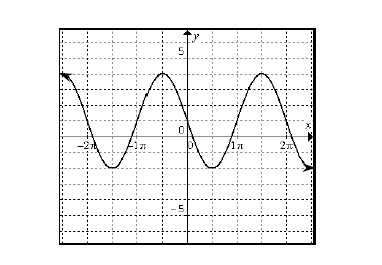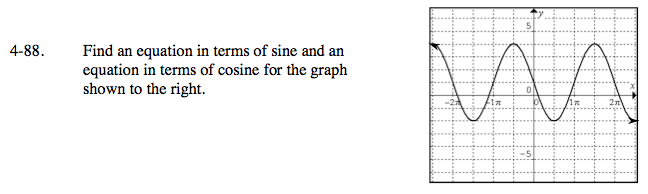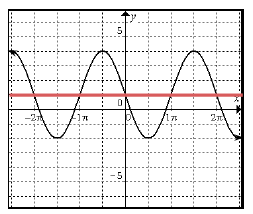### Home > PC > Chapter 4 > Lesson 4.2.2 > Problem4-88

4-88.

Find an equation in terms of sine and an equation in terms of cosine for the graph shown below. Homework Help ✎Find the mid-line of the graph.What is the vertical shift?

What is the amplitude?

What is the easiest shift for the sine?

What is the easiest shift for the cosine?

+1

+3

Flip it upside down.

Horizontal shift to the left π/2.

Use the eTool below to visualize this problem.
Click on the link to the right to view the full version of the eTool: PCT 4-88 eTool